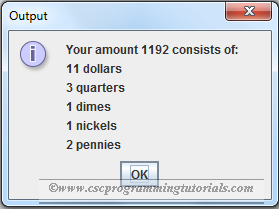# Exercise 2.26: Use Input Dialog

The program below is the solution to Liang's Introduction to Java Programming (9th Edition) Chapter 2 Exercise 2.26.

Question: Rewrite Listing 2.10, ComputeChange.java, using input and output dialog boxes.

```/**
*
* @Author: Aghatise Osazuwa
* Website: www.cscprogrammingtutorials.com
*
* Exercise 2.26 - Use input dialog
*
*/

import javax.swing.JOptionPane;

public class Ex02_26 {

public static void main(String[] args) {

String sAmount = JOptionPane.showInputDialog(null, "Enter an amount, for"
+ " example, 1156 for \$11.56: ","Input", JOptionPane.QUESTION_MESSAGE);
int amount = Integer.parseInt(sAmount);
int remainingAmount = amount;
int numberOfOneDollars = remainingAmount / 100;
remainingAmount = remainingAmount % 100;
// Find the number of quarters in the remaining amount
int numberOfQuarters = remainingAmount / 25;
remainingAmount = remainingAmount % 25;
// Find the number of dimes in the remaining amount
int numberOfDimes = remainingAmount / 10;
remainingAmount = remainingAmount % 10;
// Find the number of nickels in the remaining amount
int numberOfNickels = remainingAmount / 5;
remainingAmount = remainingAmount % 5;
// Find the number of pennies in the remaining amount
int numberOfPennies = remainingAmount;
// Display results
String output = "Your amount " + amount + " consists of: \n"
+ numberOfOneDollars + " dollars\n"
+ numberOfQuarters + " quarters\n"
+ numberOfDimes + " dimes\n"
+ numberOfNickels + " nickels\n"
+ numberOfPennies + " pennies";
JOptionPane.showMessageDialog(null, output, "Output", 1);
}
}
```Program output
Click here to see other solutions to Introduction to Java Programming.